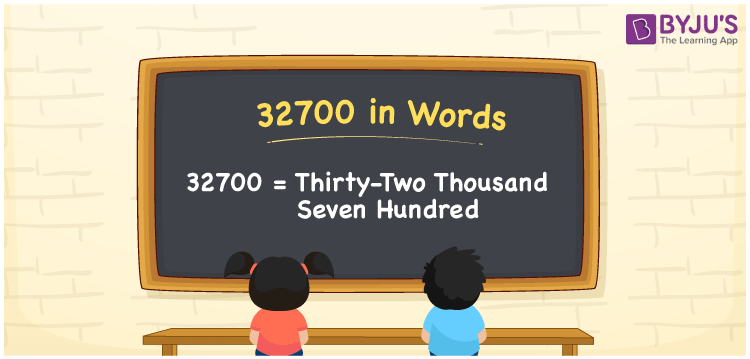# 32700 in Words

The number 32700 in words is written as Thirty-Two Thousand Seven Hundred. For example, if you bought a refrigerator worth Rs. 32700, then you can say, “I bought a refrigerator worth Rupees Thirty-Two Thousand Seven Hundred”. We know that 32700 is a cardinal number as it shows some quantity. Learn how to spell and write the number 32700 in English in this article.

 32700 in Words Thirty-Two Thousand Seven Hundred Thirty-Two Thousand Seven Hundred in numerical form 32700

## 32700 in English Words

Generally, we express numbers in words using the English alphabet. Hence, we can read the number 32700 in English as Thirty-Two Thousand Seven Hundred.## How to Write 32700 in Words?

Place value chart is important to write the number 32700 in words. So, let us create a table of 5 columns to represent the place value chart for the number 32700 as it is a five-digit number.

 Ten Thousands Thousands Hundreds Tens Ones 3 2 7 0 0

Thus, we can write the expanded form as:

3 x Ten Thousand + 2 x Thousand + 7 x Hundred + 0 x Ten + 0 x One

= 3 x 10000 + 2 x 1000 + 7 x 100 + 0 x 10 + 0 x 1

= 30000 + 2000 + 700 + 0 + 0

= 30000 + 2000 + 700

= 32700

= Thirty-Two Thousand Seven Hundred

Therefore, 32700 in words is written as Thirty-Two Thousand Seven Hundred

Interesting way of writing 32700 in words

3 = Three

32 = Thirty-Two

327 = Three Hundred and Twenty-Seven

3270 = Three Thousand Two Hundred Seventy

32700 =Thirty-Two Thousand Seven Hundred

Thus, the word form of the number 32700 is Thirty-Two Thousand Seven Hundred

32700 is a natural number that is the successor of 32699 and the predecessor of 32701

• 32700 in words – Thirty-Two Thousand Seven Hundred
• Is 32700 an odd number? – No
• Is 32700 an even number? – Yes
• Is 32700 a perfect square number? – No
• Is 32700 a perfect cube number? – No
• Is 32700 a prime number? – No
• Is 32700 a composite number? – Yes

## Frequently Asked Questions on 32700 in Words

Q1

### Write 32700 in words.

32700 in words is written as Thirty-Two Thousand Seven Hundred.
Q2

### Simplify 42700 – 10000, and express in words.

Simplifying 42700 – 10000, we get 32700. Hence, 32700 in words is Thirty-Two Thousand Seven Hundred.
Q3

### 32700 is an odd number. True or False.

False, 32700 is not an odd number.